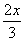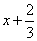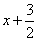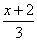1. 5 decreased by x x - 5 x + 5 5 - x 5 + x 2. Write using symbols: the sum of x and 2,divided by 33. Write using symbols: twice x 2x 2 + x 2 - x x2 4. Write using symbols: the quotient of the difference of x and y and 5 less than y(x - y)(y - 5) (x - y)(5 - y) 5. The correct exponential form of 2(x)(x)(y)(y)(y) is ? 2x3y2 2x (3y) 2(xy2)3 2x2y3 6. The correct exponential form of 3(a)(a)(a)(a)(b)(b)(c) is ? 3a4b2c 3a3b2c2 3 + 4a +2b + c 3a4b3c 7. (3x)3 in expanded form is ? 27x (3x)(3x)(3x) 3(x)(x)(x) 9x 8. (3x)2y3 in expanded form is (3x)(3x)(y)(y)(y) (3x)(3x)(3x)(y)(y) 9(x)(x)(y)(y)(y) 3(x)(x)(y)(y)(y) 9. Evaluate if x = 2: (3x)3 36 216 18 24 10. Write using exponents: (a)(a) + (b)(b)(b) (a + b)5 a2b3 a2 + b3 2a + 3b